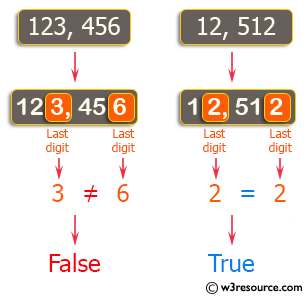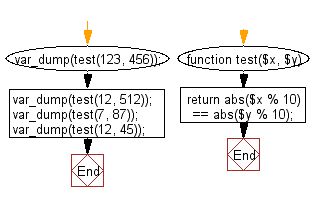﻿ PHP Exercises: Check if two given non-negative integers have the same last digit - w3resource# PHP Exercises: Check if two given non-negative integers have the same last digit

## PHP Basic Algorithm: Exercise-23 with Solution

Write a PHP program to check if two given non-negative integers have the same last digit.

Sample Solution:

PHP Code :

``````<?php
function test(\$x, \$y)
{
return abs(\$x % 10) == abs(\$y % 10);
}

var_dump(test(123, 456));
var_dump(test(12, 512));
var_dump(test(7, 87));
var_dump(test(12, 45));
``````

Sample Output:

```bool(false)
bool(true)
bool(true)
bool(false)
```

Pictorial Presentation:Flowchart:PHP Code Editor:

What is the difficulty level of this exercise?

﻿

## PHP: Tips of the Day

\$_REQUEST: This SuperGlobal Variable is used to collect data submitted by a HTML Form

Example:

This code save in a php file and run in the browser.

```<!DOCTYPE html>
<html>
<body>

<form method="post" action="<?php echo \$_SERVER['PHP_SELF'];?>">
NAME: <input type="text" name="user">
<button type="submit">SUBMIT</button>
</form>
<?php
if (\$_SERVER["REQUEST_METHOD"] == "POST") {
\$name = htmlspecialchars(\$_REQUEST['user']);
if(empty(\$name)){
echo "Name is empty";
} else {
echo \$name;
}
}
?>
</body>
</html>
```

Output:

```Owen
```